Desirable Properties & List of Tests: Aggregates - 1

# Desirable Properties & List of Tests: Aggregates - 1 - Notes | Study Transportation Engineering - Civil Engineering (CE)

 1 Crore+ students have signed up on EduRev. Have you?

Shape Test
Aim To determine the Flakiness Index, Elongation Index and Angularity Number of the given sample of aggregate.

Theory
The particle shape of aggregate is determined by percentages of flaky and elongated particles contained in it. In case of gravel it is determined by its angularity number. For base course and construction of bituminous and cement concrete types, the presence of flaky and elongated particles are considered undesirable as they may cause inherent weakness with possibilities of breaking down under heavy loads. Rounded aggregate are preferred in cement concrete road construction as the workability of concrete improves. Angular shape of particles is desirable for granular base course due to increased stability derived from the better interlocking. Thus evaluation of shape of the particles, particularly with reference to flakiness, elongation and angularity is necessary.
1. Flakiness Index
The Flakiness index of aggregate is the percentage by weight of particles whose least dimension [thickness] is less than three- fifths [0.6] times of their mean dimension. The test is not applicable to aggregate size smaller than 6.3 mm
Apparatus
a) Thickness gauge
b) Weighing balance
c) IS Sieves of sizes 63 mm, 50 mm, 40 mm, 31.5 mm,25 mm,20 mm, 16 mm, 12.5 mm, 10 mm and 6.3 mm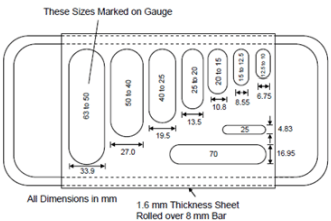Procedure
1. The sieves are arranged such that the largest size sieve (63 mm) is at the top and the smallest size sieve (6.3 mm) is at the bottom.
2. The given aggregate are sieved. A minimum of 200 pieces of each fraction to be tested are taken and weighed = W1 g.
3. In order to separate flaky aggregate, each fraction is then gauged for thickness through the respective opening on the thickness gauge. For example, if the aggregate is from 50 – 40 fraction, it is gauged through the opening of 27.00 mm on the thickness gauge.
4. The flaky aggregate passing the respective openings are collected and accurately weighed = w1 g.
5. The procedure is repeated for other fractions having weights W2, W3, etc. and the flaky aggregate in them having weights w2, w3 respectively are weighed.
6. Then
Flakiness Index =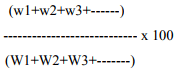Result
The flakiness Index of the given sample of aggregate is __________
2. Elongation Index
The elongation index of an aggregate is the percentage by weight of particles whose greatest dimension (length) is greater than one and four fifth times [1. 8] times their mean dimension. The elongation test is not applicable to size smaller than 6.3 mm.
Apparatus
a) Length gauge
b) Weighing balance
c) IS Sieve of size as in flakiness Index test.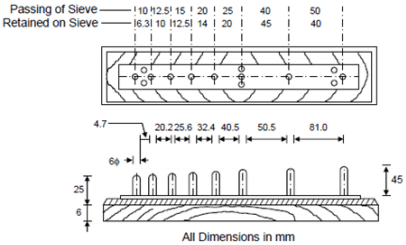Procedure
1. The sample is sieved through the IS sieves specified and a minimum of 200 pieces of each fraction are taken and weighed = W1 g.
2. In order to separate elongated aggregate , each fraction is then gauged individually for length through the respective opening on the length gauge
3. In each fraction, the aggregate retained on the respective opening on the length gauge is collected and weighed =X1 g.
4. The procedure is repeated for other fractions having weights W2, W3, etc, and the weights of elongated aggregate in them X2, X 3 etc, are found.
5. Then

Elongation Index =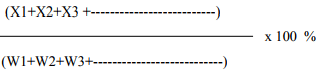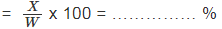Result The elongation Index of the given sample of aggregate = __________ %

3. Angularity Number
The angularity number of an aggregate is the amount by which the percentage voids exceeds 33, after being compacted in a prescribed manner. The angularity number is found from the expression (67 - 100 * W / CG, percent). Here the value 67 represents the percentage volume of solids of most rounded gravel, which would have 33 percent voids
Apparatus
a) A metal cylinder closed at one end having 3 litre capacity, diameter and height approximately equal
b) A metal tamping rod, 16 mm in diameter and 600 mm long.
c) Weighing balance
d) IS sieves 25, 20, 16,12.5, 10, 6.3 and 4.75 mm

Procedure
1) The sieves for each fraction (as specified) are arranged such as 25 - 20 mm, etc.
2) The given sample of aggregate is sieved so that sufficient pieces are obtained in each fraction.
3) The empty cylinder is accurately weighed = „ a‟ g
4) Each aggregate fraction is separately filled in the cylinder in 3 (three) layers tamping each layer 100 (hundred) times with the rounded end of tamping rod. The excess aggregate are removed.
5) The cylinder along with aggregate is weights = „b‟ g
6) The aggregate are removed from the cylinder. The cylinder is completely filled with water and after wiping its outer sides it is weighed with water = „d‟ g
7) Then Angularity number may be calculated from the expression, Angularity number = 67 - 100 W / CG
Where, W= Mean weight of aggregate in the cylinder
C = Weight of water required to fill the cylinder
G= Average Specific gravity of aggregate.

Result
The angularity number of the given sample of aggregate = …………. %
Observations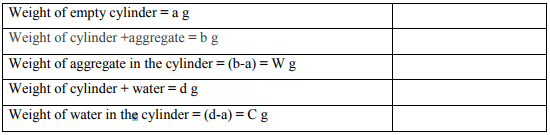Flakiness Index and Elongation Index Observations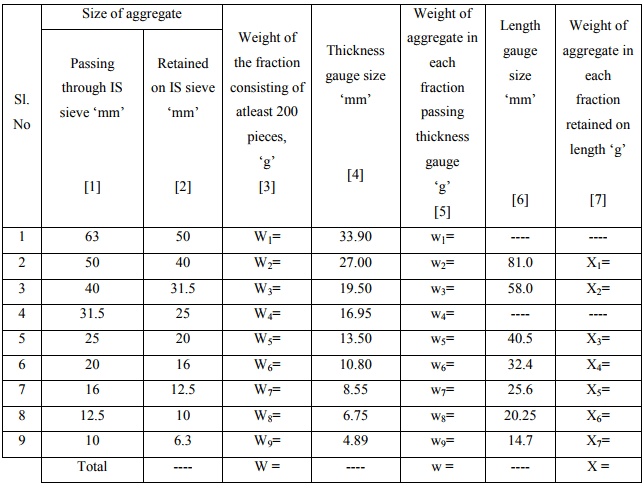Flakiness Index =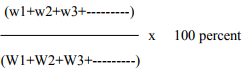Elongation Index =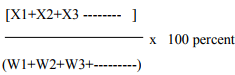Angularity Number = 67 – 100 W/ CG

Tabular Column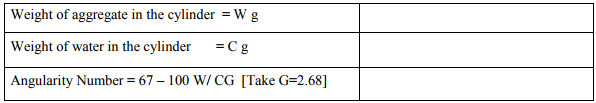Desirable value
IRC has recommended the maximum allowable limits of flakiness index values for various types of construction are given below: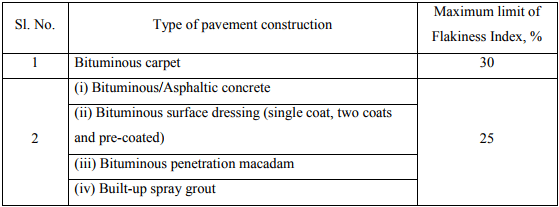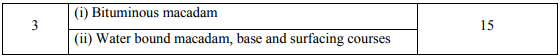Though elongated shape of the aggregates also affects the compaction and the construction of pavements, there are no specified limits of elongation index values as in the case of flakiness index for different methods of pavement construction.
The angularity number measures the percent voids in excess of 33 percent which is obtained in the case of the most rounded gravel particles. The angularity number of aggregates generally ranges from zero for highly rounded gravel to about 11 for freshly crushed angular aggregates. Slightly higher values of angularity number also may be obtained in the case of highly angular and flaky aggregates. Thus higher the angularity number, more angular and less workable is the aggregate mix.
It is desirable that the flakiness index of aggregates used in road construction is less than 15% and normally does not exceed 25%.
Elongation index value in excess of 15% percent is considered undesirable, however no recognized limits have been laid down for elongation index.

The document Desirable Properties & List of Tests: Aggregates - 1 - Notes | Study Transportation Engineering - Civil Engineering (CE) is a part of the Civil Engineering (CE) Course Transportation Engineering.
All you need of Civil Engineering (CE) at this link: Civil Engineering (CE)

## Transportation Engineering

22 videos|68 docs|58 tests

## Transportation Engineering

22 videos|68 docs|58 tests

Track your progress, build streaks, highlight & save important lessons and more!

,

,

,

,

,

,

,

,

,

,

,

,

,

,

,

,

,

,

,

,

,

;